# Index Section A Section B Section C Section

• Slides: 49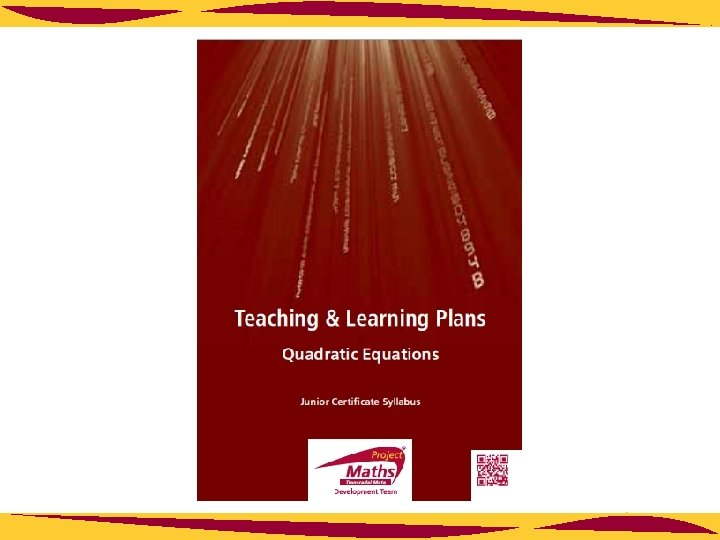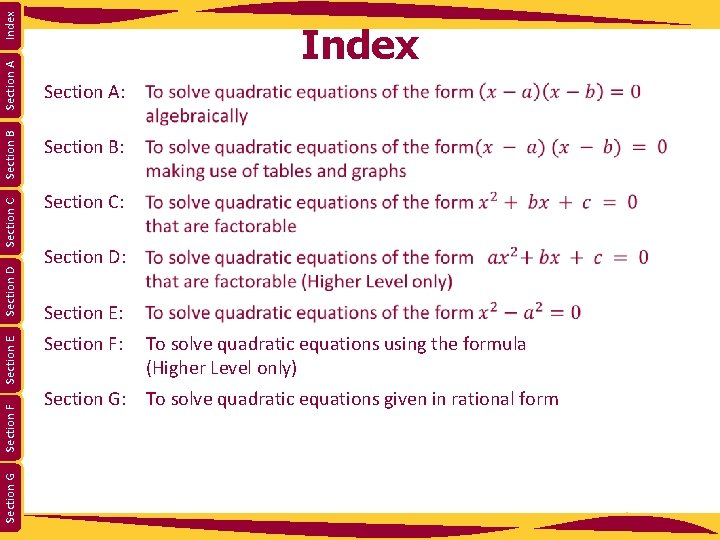Index Section A Section B Section C Section D Section E Section F Section G Index Section A: Section B: Section C: Section D: Section E: Section F: To solve quadratic equations using the formula (Higher Level only) Section G: To solve quadratic equations given in rational form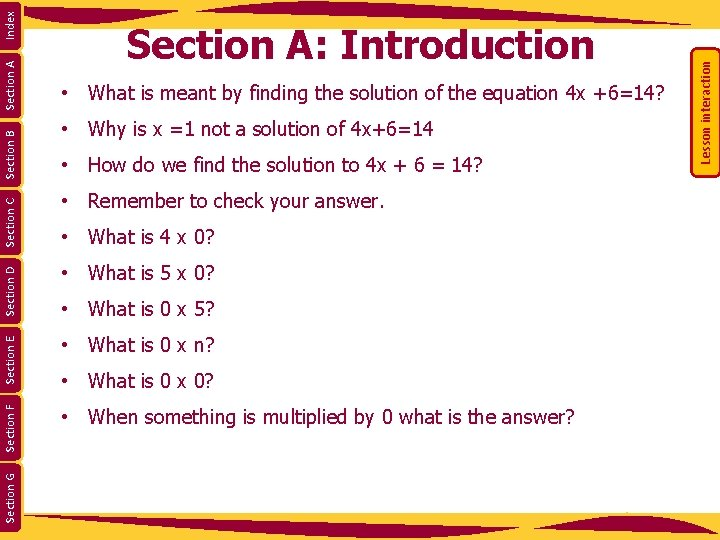• Why is x =1 not a solution of 4 x+6=14 • How do we find the solution to 4 x + 6 = 14? • Remember to check your answer. • What is 4 x 0? • What is 5 x 0? • What is 0 x n? • When something is multiplied by 0 what is the answer? Section G Section E • What is 0 x 5? • What is 0 x 0? Lesson interaction Index Section A Section B Section C • What is meant by finding the solution of the equation 4 x +6=14? Section F Section D Section A: Introduction• Compare (x - 3)(x - 4) = 0, with xy = 0 and what information can we arrive at? • If x - 3 = 0, what does this tell us about x? • If x - 4 = 0, what does this tell us about y? • How do we check if x = 3 is a solution to (x - 3)(x - 4) = 0? • Write in your copies in words what x = 3 or x= 4 means in the • When an equation is written in the form (x - a)(x - b)=0, what Section G Section E • How do we check if x = 4 is a solution to (x - 3)(x - 4) =0? context of (x - 3)(x - 4) = 0. are the solutions? Lesson interaction Index Section A Section B Section C • If xy = 0 what do we know about x and or y? Section F Section D Section A: Introduction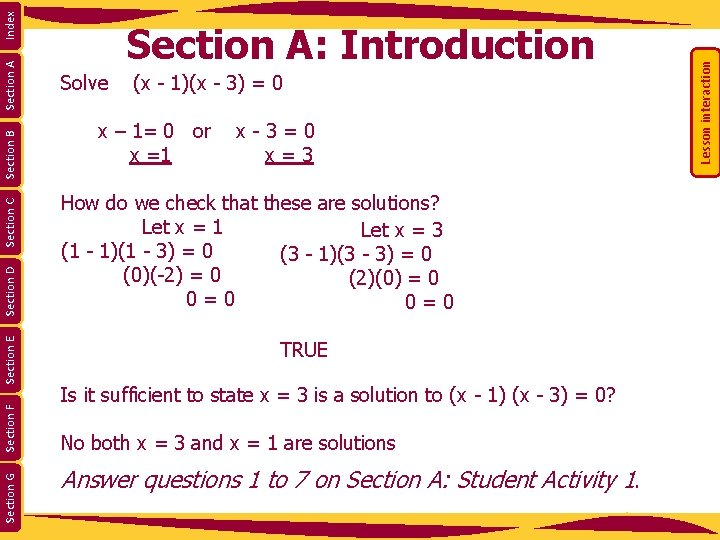Solve (x - 1)(x - 3) = 0 x – 1= 0 or x - 3 = 0 x =1 x = 3 How do we check that these are solutions? Let x = 1 Let x = 3 (1 - 1)(1 - 3) = 0 (3 - 1)(3 - 3) = 0 (0)(-2) = 0 (2)(0) = 0 0 = 0 TRUE Is it sufficient to state x = 3 is a solution to (x - 1) (x - 3) = 0? No both x = 3 and x = 1 are solutions Answer questions 1 to 7 on Section A: Student Activity 1. Lesson interaction Index Section A Section B Section C Section D Section E Section F Section G Section A: Introduction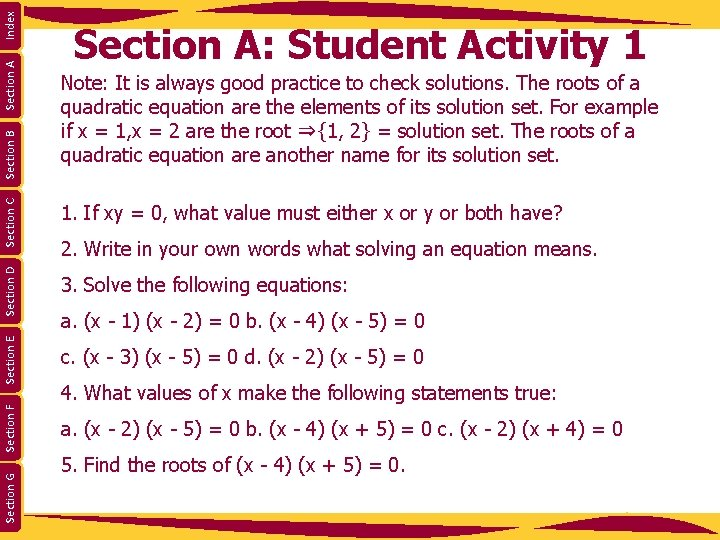Index Section A Section C Note: It is always good practice to check solutions. The roots of a quadratic equation are the elements of its solution set. For example if x = 1, x = 2 are the root ⇒{1, 2} = solution set. The roots of a quadratic equation are another name for its solution set. 1. If xy = 0, what value must either x or y or both have? Section D Section B Section A: Student Activity 1 3. Solve the following equations: 2. Write in your own words what solving an equation means. Section E a. (x - 2) (x - 5) = 0 b. (x - 4) (x + 5) = 0 c. (x - 2) (x + 4) = 0 Section G c. (x - 3) (x - 5) = 0 d. (x - 2) (x - 5) = 0 Section F a. (x - 1) (x - 2) = 0 b. (x - 4) (x - 5) = 0 4. What values of x make the following statements true: 5. Find the roots of (x - 4) (x + 5) = 0.Index Section A Hence state what the roots of (x - 3) (x + 2) = 0 are. 6. Solve the equation (x - 3) (x + 2) = 0. 7. Find a positive value for x that makes the statement (x - 4) (x + 2) = 0 true. a. x (x - 1) = 0 each case: Section G Section F Section D 8. Solve the following equations: Section E Section C 5. Find the roots of (x - 4) (x + 5) = 0. Section B Section A: Student Activity 1 b. x (x - 2) = 0 c. x (x + 4) = 0 9. a. These students each made at least one error, explain the error(s) in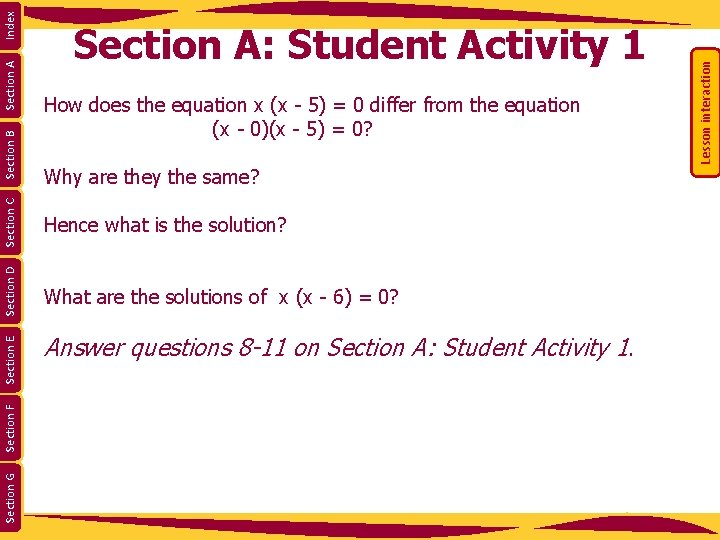How does the equation x (x - 5) = 0 differ from the equation (x - 0)(x - 5) = 0? Why are they the same? Hence what is the solution? What are the solutions of x (x - 6) = 0? Answer questions 8 -11 on Section A: Student Activity 1. Lesson interaction Index Section A Section B Section C Section D Section E Section F Section G Section A: Student Activity 1Index Section A 9. a. These students each made at least one error, explain the error(s) in Section G Section F Section E Section D Section C 8. Solve the following equations: Section B Section A: Student Activity 1 a. x (x - 1) = 0 each case: b. x (x - 2) = 0 c. x (x + 4) = 0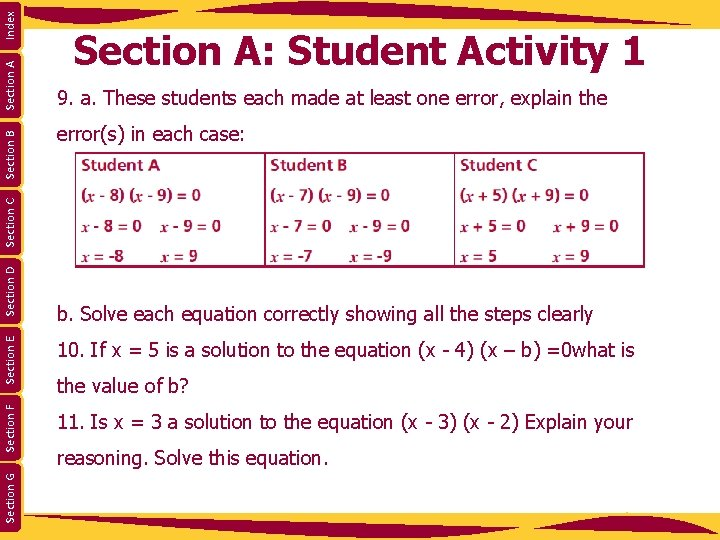Index Section A 9. a. These students each made at least one error, explain the error(s) in each case: 10. If x = 5 is a solution to the equation (x - 4) (x – b) =0 what is 11. Is x = 3 a solution to the equation (x - 3) (x - 2) Explain your Section G Section E b. Solve each equation correctly showing all the steps clearly Section F Section D Section C Section B Section A: Student Activity 1 the value of b? reasoning. Solve this equation.Section G Section F Section E Section D Section C Section A Lesson interaction Section B Index Section B: Introduction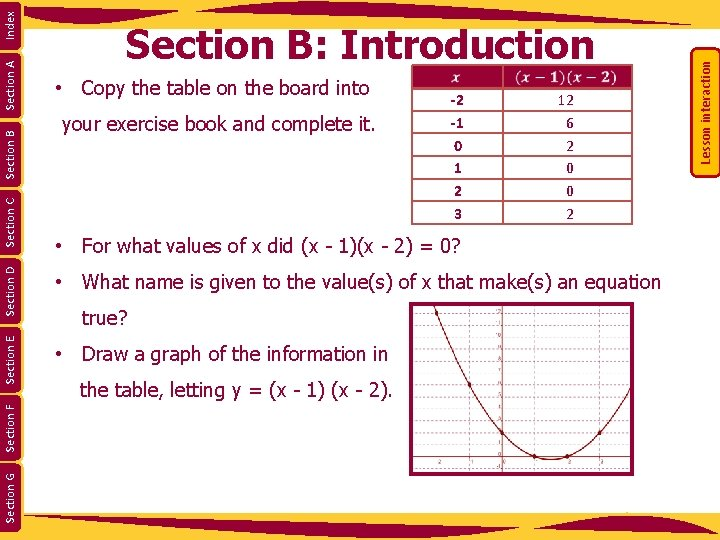your exercise book and complete it. -2 -1 0 1 2 3 12 6 2 0 0 2 • What name is given to the value(s) of x that make(s) an equation • Draw a graph of the information in Section G Section F Section D • For what values of x did (x - 1)(x - 2) = 0? true? the table, letting y = (x - 1) (x - 2). Lesson interaction Index Section A Section B • Copy the table on the board into Section E Section C Section B: IntroductionLesson interaction • For what values of x did the graph cut the x axis? • What is meant by the solution of an equation? • What was the value of y =(x - 1)(x - 2) when x = 1 and x = 2? Lesson interaction Index Section A Section B Section C Section D Section E Section F Section G Section B: Introduction • When using algebra to solve, what were the values of x for which (x - 1) (x - 2) = 0 called? • How can we get the solution by looking at the table? • How can we get the solution by looking at the graph? Complete the exercises in Section B: Student Activity 2. -2 -1 0 1 2 3 12 6 2 0 0 21. a. Complete the following table: Index Section G Section F Section E Section D Section C Section B Section A Section B: Student Activity 2 b. From the table above determine the values of x for which the equation is equal to 0. c. Solve the equation (x + 2) (x + 1) = 0 by algebra. d. What do you notice about the answer you got to parts b. and c. in this question? e. Draw a graph of the data represented in the above table. f. Where does the graph cut the x axis? What is the value of f (x) = (x + 2)(x + 1) at the points where the graph cuts the x axis? g. Can you describe three methods of finding the solution to (x + 2) (x + 1) = 0.Index Section A Section B Section C Section D Section E Section F Section G Section B: Student Activity 2 2. Solve the equation (x - 1) (x - 4) = 0 a) by table, b) by graph and c) algebraically. 3. Write the equation represented in this table in the form (x−a)(x−b)=0.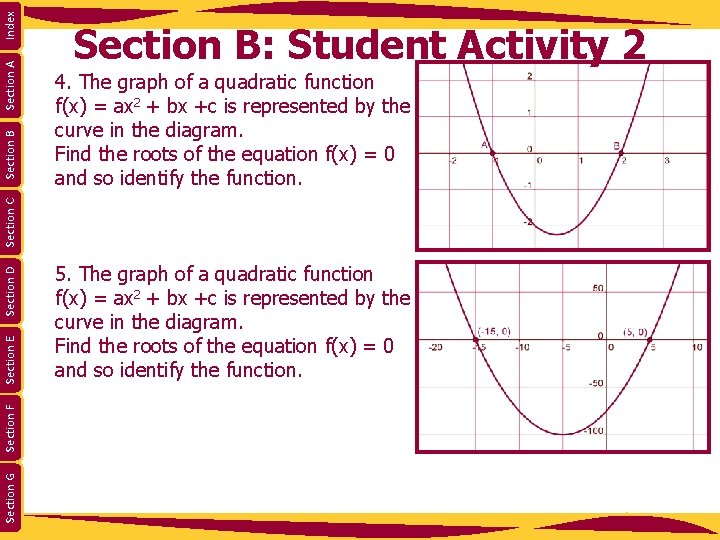Index Section A 4. The graph of a quadratic function f(x) = ax 2 + bx +c is represented by the curve in the diagram. Find the roots of the equation f(x) = 0 and so identify the function. Section G Section F Section E Section D Section C Section B: Student Activity 2 5. The graph of a quadratic function f(x) = ax 2 + bx +c is represented by the curve in the diagram. Find the roots of the equation f(x) = 0 and so identify the function.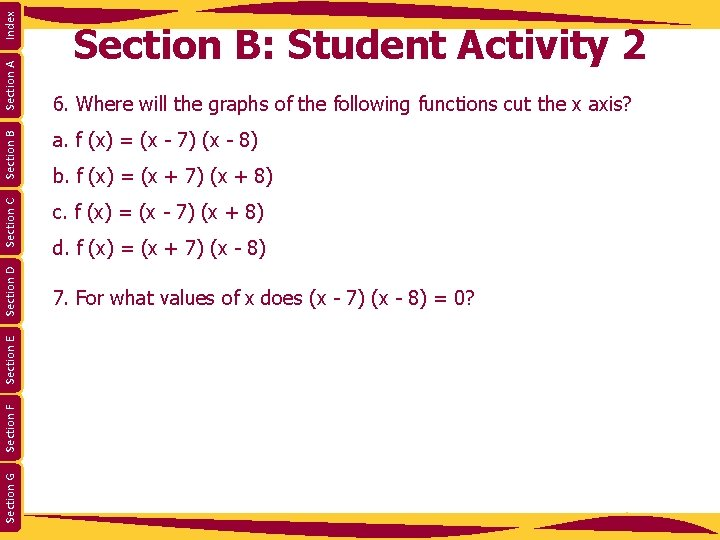Index a. f (x) = (x - 7) (x - 8) c. f (x) = (x - 7) (x + 8) Section G Section F Section E Section D Section B 6. Where will the graphs of the following functions cut the x axis? Section C Section A Section B: Student Activity 2 b. f (x) = (x + 7) (x + 8) d. f (x) = (x + 7) (x - 8) 7. For what values of x does (x - 7) (x - 8) = 0?Section G Section F Section E Section D Section C Section A Lesson interaction Section B Index Section C: IntroductionSection G Section F Section E Section D Section C Section A Lesson interaction Section B Index Section C: Introduction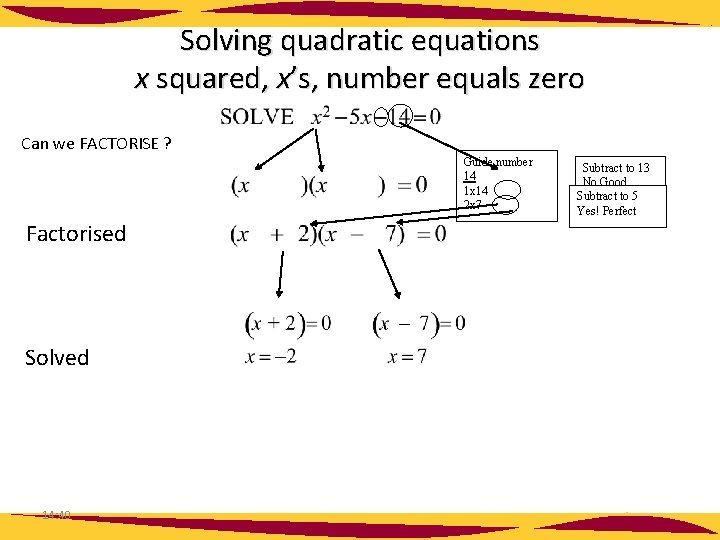Solving quadratic equations x squared, x’s, number equals zero Can we FACTORISE ? Factorised Solved 14: 40 Guide number 14 1 x 14 2 x 7 Subtract to 13 No Good Subtract to 5 Yes! Perfect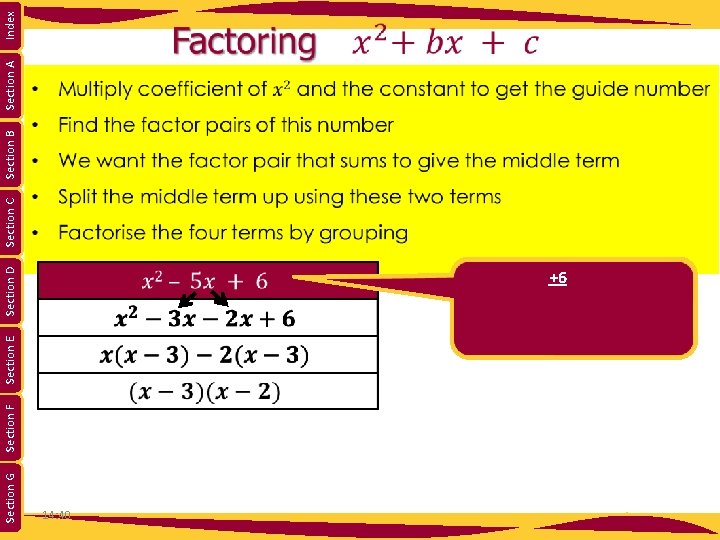Section G Section F Section E Section D Section C Section B Section A Index 14: 40 +6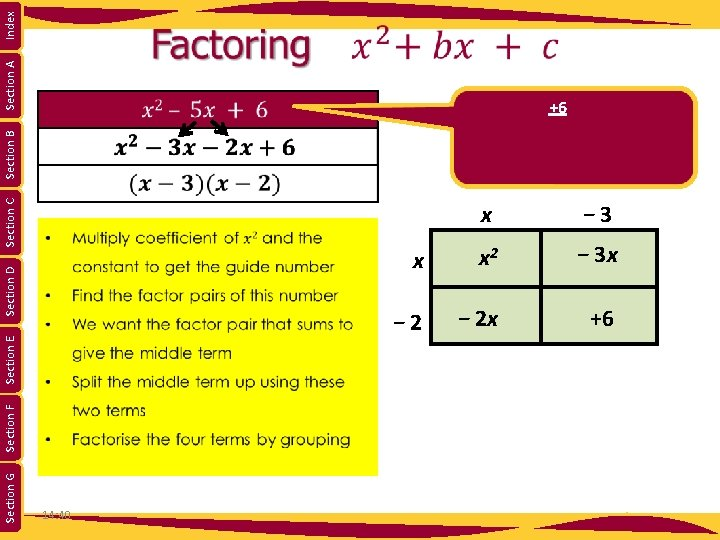Section G Section F Section E Section D Section C Section B Section A Index 14: 40 +6 x − 3 x x 2 − 3 x − 2 x +6Section G Section F Section E Section D Section C Section B Section A Index Section C: Student Activity 3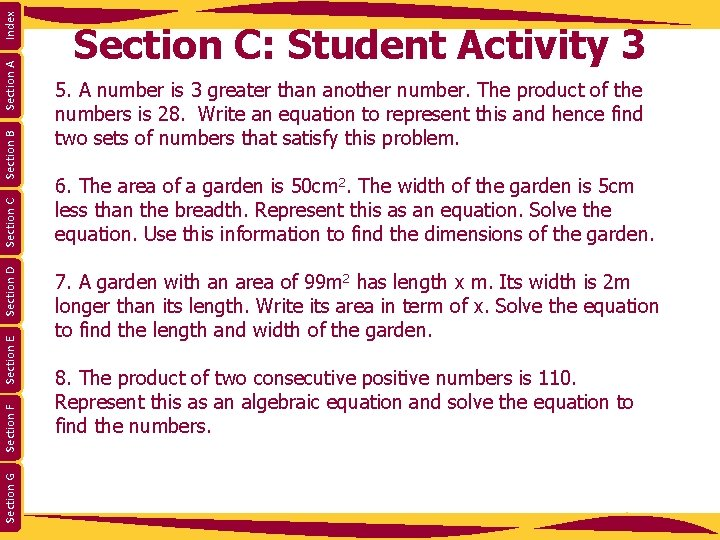Index Section A Section B Section C Section D Section E Section F Section G Section C: Student Activity 3 5. A number is 3 greater than another number. The product of the numbers is 28. Write an equation to represent this and hence find two sets of numbers that satisfy this problem. 6. The area of a garden is 50 cm 2. The width of the garden is 5 cm less than the breadth. Represent this as an equation. Solve the equation. Use this information to find the dimensions of the garden. 7. A garden with an area of 99 m 2 has length x m. Its width is 2 m longer than its length. Write its area in term of x. Solve the equation to find the length and width of the garden. 8. The product of two consecutive positive numbers is 110. Represent this as an algebraic equation and solve the equation to find the numbers.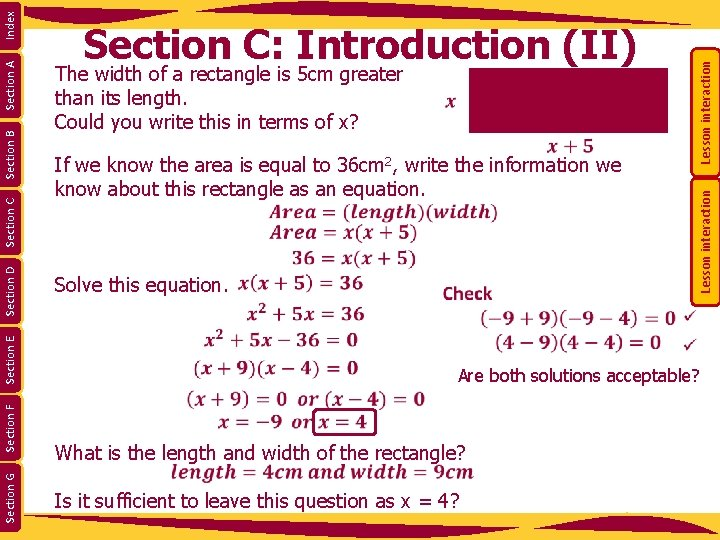Lesson interaction The width of a rectangle is 5 cm greater than its length. Could you write this in terms of x? If we know the area is equal to 36 cm 2, write the information we know about this rectangle as an equation. Lesson interaction Index Section A Section B Section C Section D Section E Section F Section G Section C: Introduction (II) Solve this equation. Are both solutions acceptable? What is the length and width of the rectangle? Is it sufficient to leave this question as x = 4?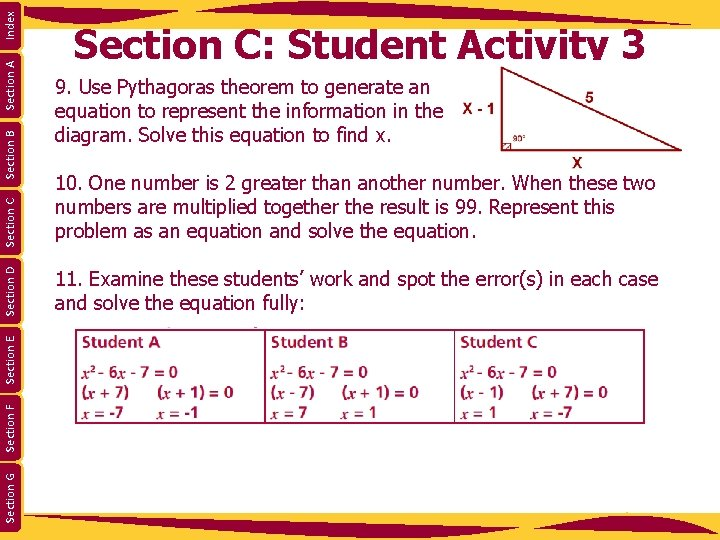Index Section A 10. One number is 2 greater than another number. When these two numbers are multiplied together the result is 99. Represent this problem as an equation and solve the equation. 11. Examine these students’ work and spot the error(s) in each case and solve the equation fully: Section G Section F Section E Section C 9. Use Pythagoras theorem to generate an equation to represent the information in the diagram. Solve this equation to find x. Section D Section B Section C: Student Activity 3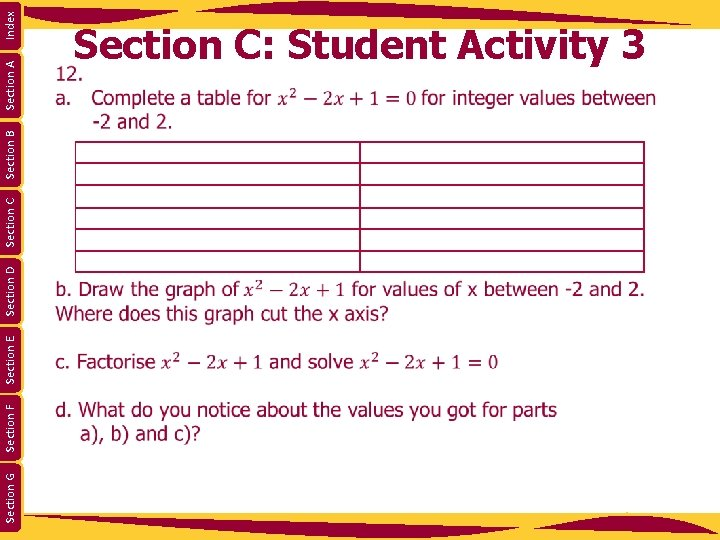Section G Section F Section E Section D Section C Section B Section A Index Section C: Student Activity 3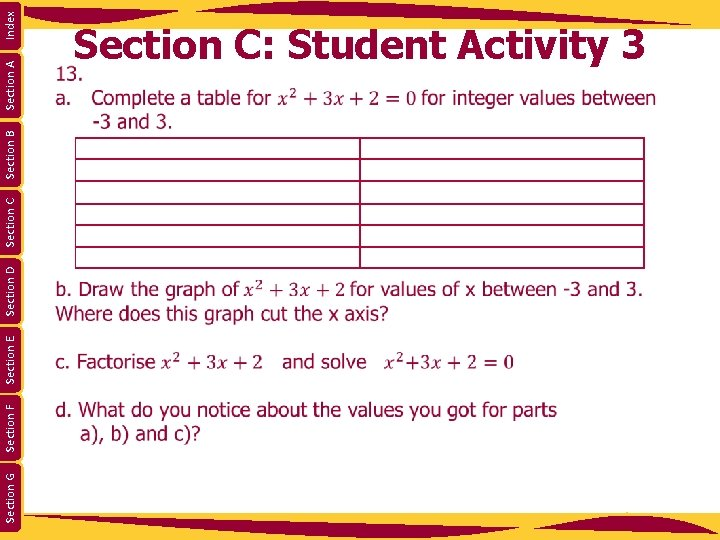Section G Section F Section E Section D Section C Section B Section A Index Section C: Student Activity 3Section G Section F Section E Section D Section C Section A Lesson interaction Section B Index Section D: IntroductionSection G Section F Section E Section D Section C Section B Section A Index 14: 42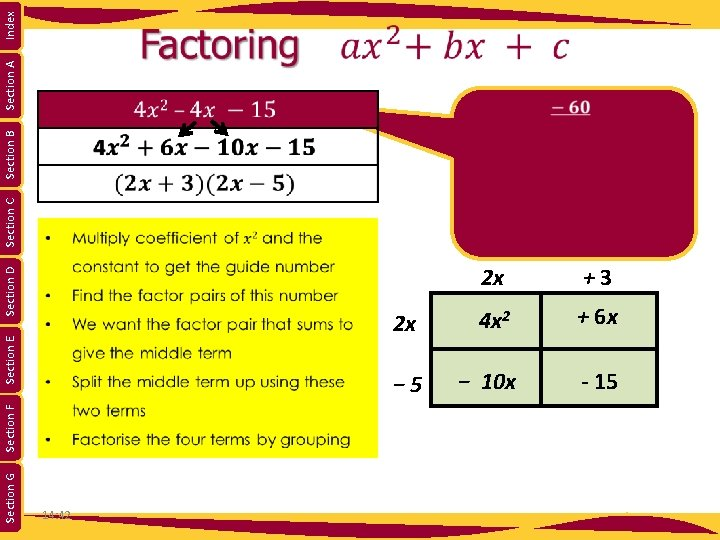Section G Section F Section E Section D Section C Section B Section A Index 14: 42 2 x +3 2 x 4 x 2 + 6 x − 5 − 10 x - 15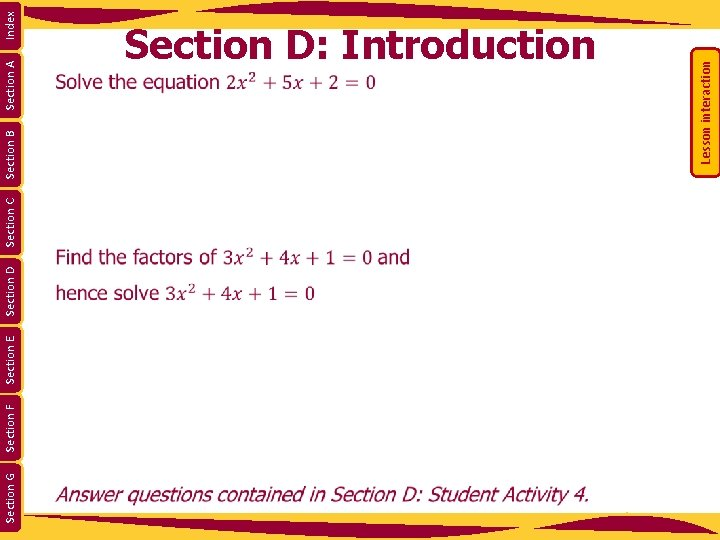Section G Section F Section E Section D Section C Section A Lesson interaction Section B Index Section D: Introduction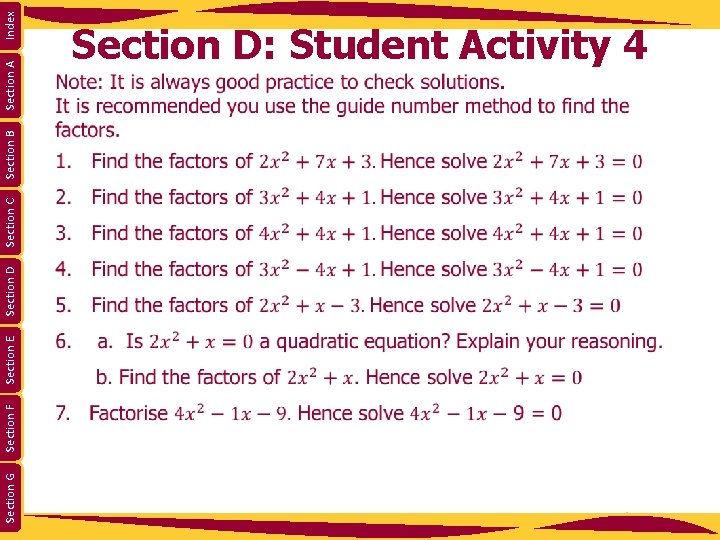Section G Section F Section E Section D Section C Section B Section A Index Section D: Student Activity 4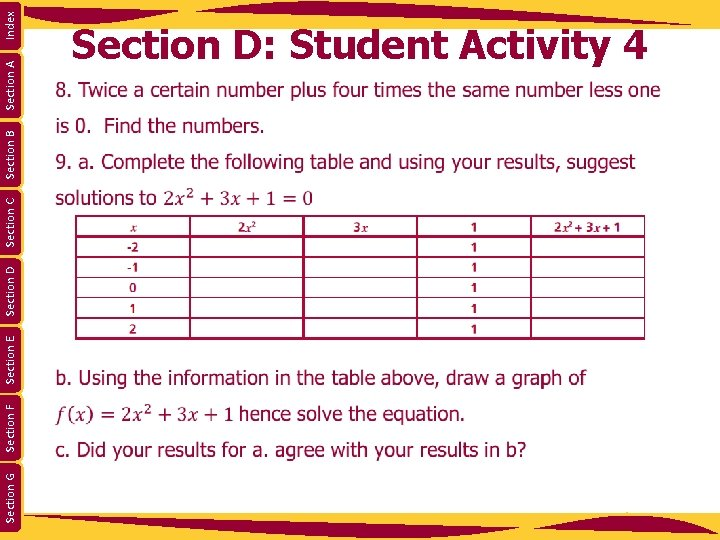Section G Section F Section E Section D Section C Section B Section A Index Section D: Student Activity 4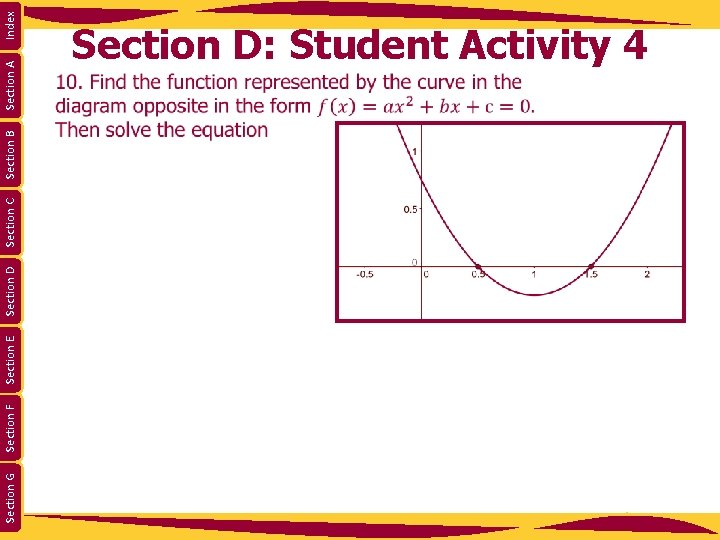Section G Section F Section E Section D Section C Section B Section A Index Section D: Student Activity 4Section G Section F Section E Section D Section C Section A Index Section E: Introduction Lesson interaction Section BSection G Section F Section E Section D Section C Section A Index Section E: Introduction Lesson interaction Section Bx Ax 2 y B x -y y 2 y x Index Section A Section G Section F Section E Section D Section C y Area A = (x) (x - y) Area B = (y) (x - y) Area A + Area B = (x) (x - y) + (y) (x - y) x 2 – y 2 = (x - y)(x + y) Complete Section E: Student Activity 5. Lesson interaction x - y Section B Section E: Introduction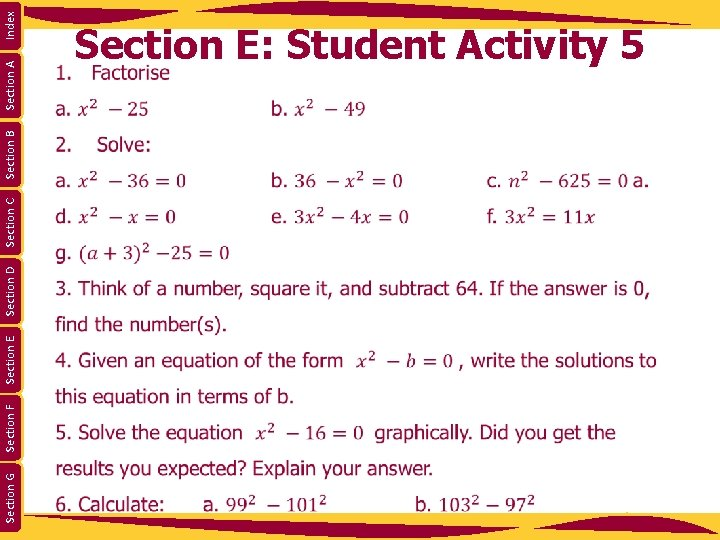Section G Section F Section E Section D Section C Section B Section A Index Section E: Student Activity 5Section G Section F Section E Section D Section C Section B Section A Index Section E: Student Activity 5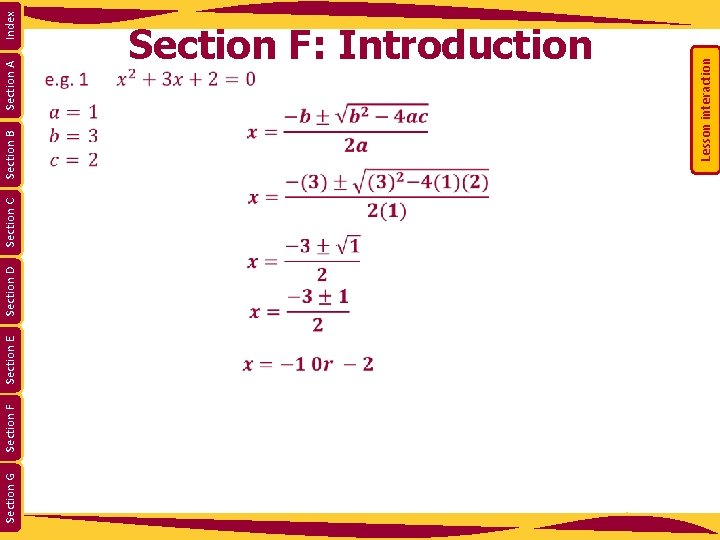Section G Section F Section E Section D Section A Lesson interaction Section B Section C Index Section F: Introduction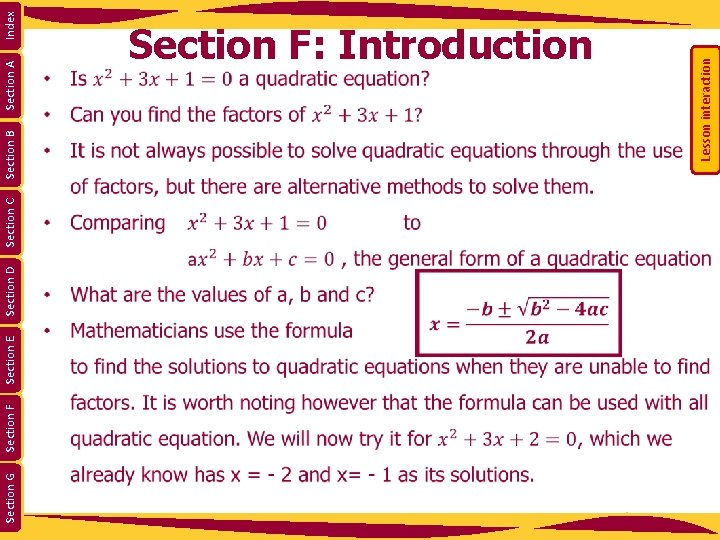Section G Section F Section E Section D Section C Section A Lesson interaction Section B Index Section F: Introduction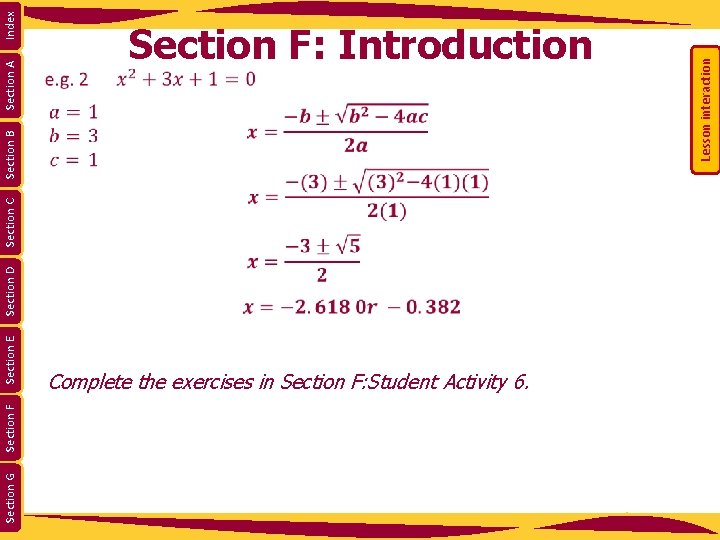Section G Section F Section E Section D Section A Complete the exercises in Section F: Student Activity 6. Lesson interaction Section B Section C Index Section F: Introduction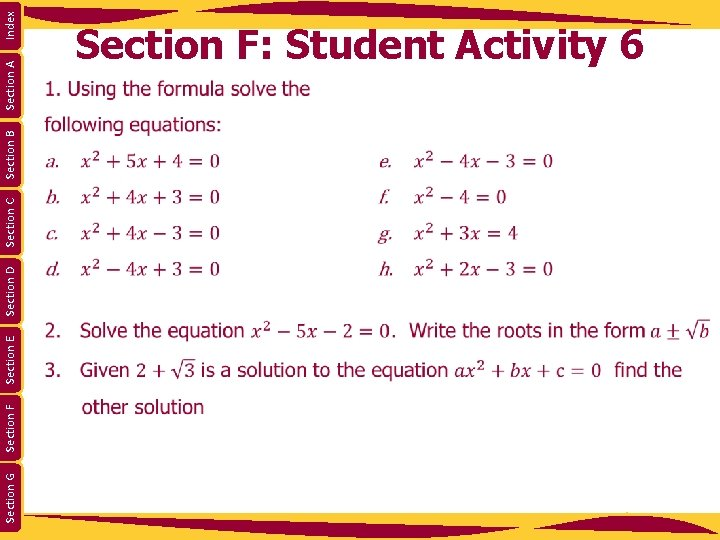Section G Section F Section E Section D Section C Section B Section A Index Section F: Student Activity 6Section G Section F Section E Section D Section C Section B Section A Index Section F: Student Activity 6Section G Section F Section E Section D Section C Section B Section A Index Lesson interaction Section G: Introduction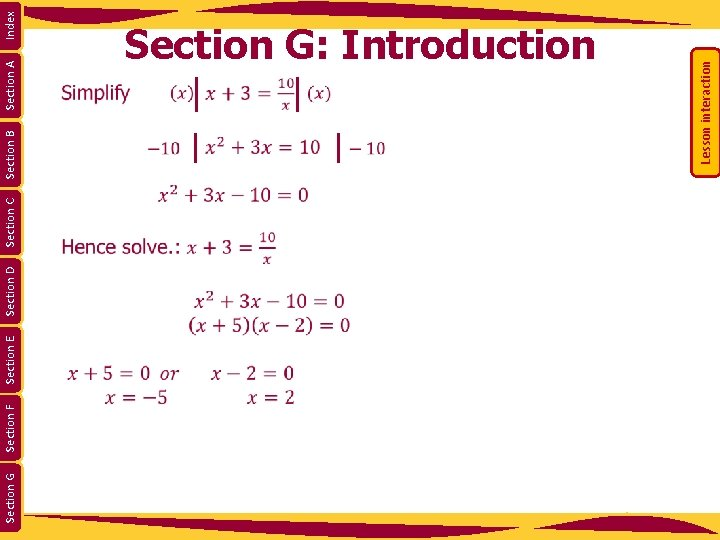Section G Section F Section E Section D Section C Section B Section A Index Lesson interaction Section G: IntroductionSection G Section F Section E Section D Section C Section B Section A Index Section G: Student Activity 7 Solve the following equations: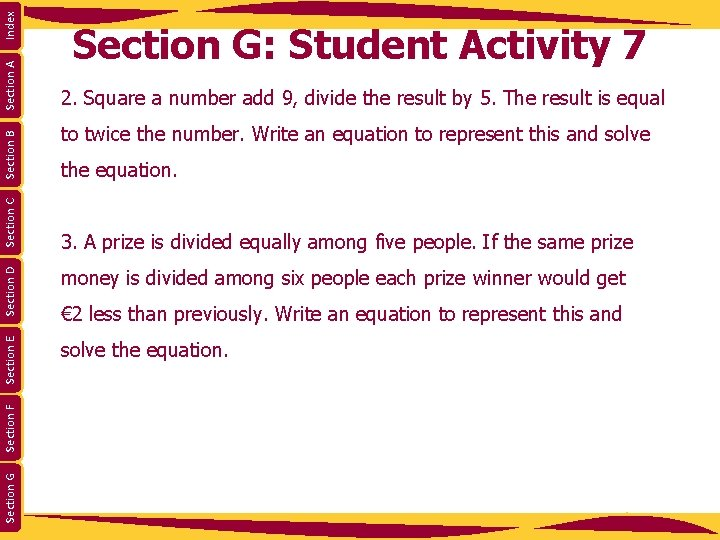Index Section A Section B 2. Square a number add 9, divide the result by 5. The result is equal to twice the number. Write an equation to represent this and solve the equation. money is divided among six people each prize winner would get solve the equation. Section G Section F Section D 3. A prize is divided equally among five people. If the same prize Section E Section C Section G: Student Activity 7 € 2 less than previously. Write an equation to represent this and